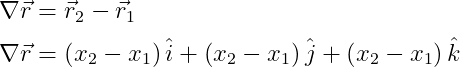# What are Vectors in Physics?

A physical quantity is a quantity whose physical properties you can measure. Such as mass, force, velocity, displacement, temperature, etc.

Suppose you are told to measure your happiness. In this case, you can never measure your happiness. That is, you cannot describe and analyze with measure how much happiness you have. So, happiness here is not a physical quantity.

Suppose you have a fever. And the doctor ordered you to measure your body temperature. Then you measured your body temperature with a thermometer and told the doctor. When you tell your doctor about your body temperature, you need to use the word degree centigrade or degree Fahrenheit.

So, the temperature here is a measurable quantity. So we will use temperature as a physical quantity.

In general, we will divide the physical quantity into three types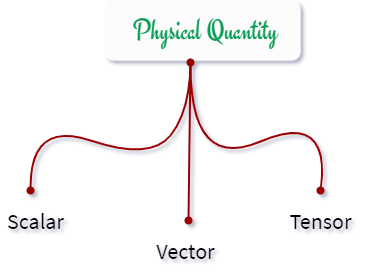In this tutorial, we will only discuss vector quantity. But before that, let’s talk about scalar.

## Scalar Quantity

We will call the scalar quantity the physical quantity which has a value but does not have a specific direction.

Suppose you are allowed to measure the mass of an object. So, you do not need to specify any direction when you determine the mass of this object. That is, mass is a scalar quantity.

There are many physical quantities like this that do not need to specify direction when specifying measurable properties. Such as temperature, speed, distance, mass, etc.

## Introduction to Vector in Physics

Simply put, vectors are those physical quantities that have values ​​as well as specific directions. Such as displacement, velocity, etc.

That is, you need to describe the direction of the quantity with the measurable properties of the physical quantity here.

For example, many of you say that the velocity of a particle is five. Since velocity is a vector quantity, just mentioning the value is not a complete argument. You need to specify the direction along with the value of velocity. So, you have to say that the value of velocity in the specified direction is five.

Graphically, a vector is represented by an arrow. Here, the vector is represented by ab. That is, the value of the given vector will depend on the length of the ab vector. And a is the initial point and b is the final point.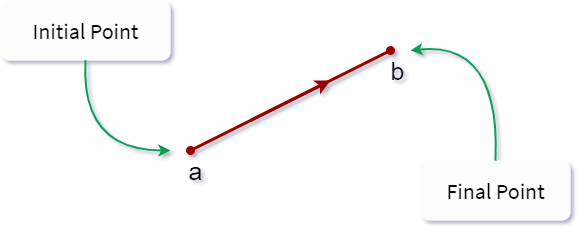Analytically, a vector is represented by an arrow above the letter. And the value of the vector is always denoted by the mod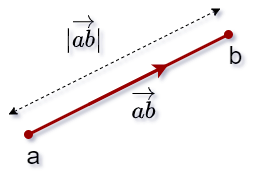## Types of Vectors in Physics

We can divide the vector into different types according to the direction, value, and position of the vector. For example

### 1. Equal Vectors

When two or more vectors have equal values ​​and directions, they are called equal vectors. That is, the initial and final points of each vector may be different. But, the direction can always be the same. So, look at the figure below, here are three vectors are taken.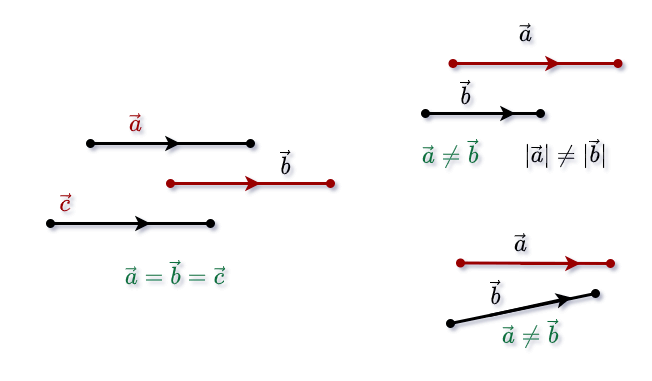### 2. Opposite Vectors

Absolute values ​​of two vectors are equal but when the direction is opposite they are called opposite vectors. That is, here the absolute values ​​of the two vectors will be equal but the two vectors will be at a degree angle to each other.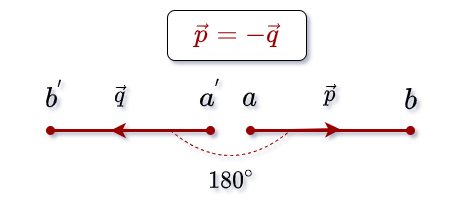### 3. Collinear Vectors

When multiple vectors are located along the same parallel line they are called collinear vectors. In this case, the value and direction of each vector may be the same and may not be the same. However, the direction of each vector will be parallel.

That is, each vector will be at an angle of 0 degrees or 180 degrees with all other vectors. Notice in the figure below that each vector here is along the x-axis.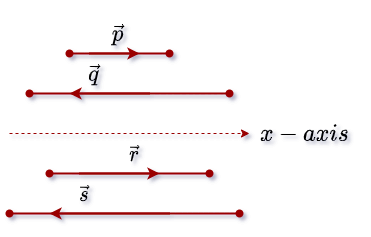Here both equal vector and opposite vector are collinear vectors.

### 4. Coplanar Vectors

When multiple vectors are located on the same plane, they are called coupler vectors. Notice below, a, b, c are on the same plane.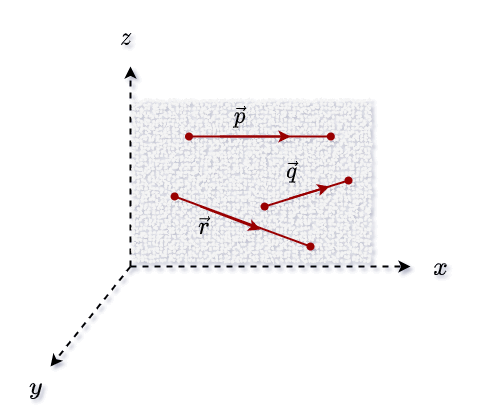### 5. Unit Vector

When the value of the vector in the specified direction is one, it is called the unit vector in that direction. That is, dividing a vector by its absolute value gives a unit vector in that direction. And, the unit vector is always a dimensionless quantity.

The absolute value of a vector is a scalar. That is, by multiplying the unit vector in the direction of that vector with that absolute value, the complete vector can be found. So, look at the figure below.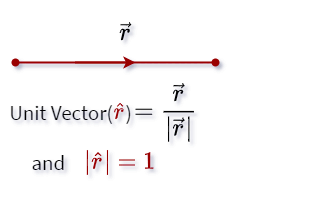Unit vectors are usually used to describe a specified direction

### 6. Null Vector

If the initial point and the final point of the directional segment of a vector are the same, then the segment becomes a point. Thus, this type of vector is called a null vector. Thus, it is a vector whose value is zero and it has no specific direction.

Suppose a particle is moving in free space. And the particle T started its journey from one point and came back to that point again i.e. displacement of the particle will be zero. Thus, since the displacement is the vector quantity. So in this case x will be the vector.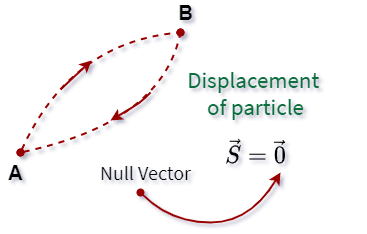Suppose again, two forces with equal and opposite directions are being applied to a particle. In this case, the total force will be zero. So, the total force will be written as zero but according to the rules of vector algebra, zero has to be represented by vectors here.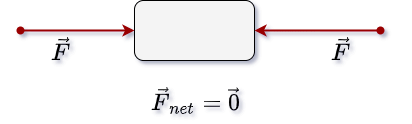When a particle moves with constant velocity in free space, the acceleration of the particle will be zero. In this case, also the acceleration is represented by the null vector.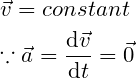## Vector Algebra in Physics

You all know that when scalar calculations are done, linear algebra rules are used to perform various operations. That is, when you do vector calculations, you have to perform different operations according to the vector algebra rule.

Vector algebra is a branch of mathematics where specific rules have been developed for performing various vector calculations. Vector calculation here means vector addition, vector subtraction, vector multiplication, and vector product. Also, equal vectors and opposite vectors are also a part of vector algebra which has been discussed earlier.

You may have many questions in your mind that what is the difference between vector algebra and linear algebra?

When you perform an operation with linear algebra, you only use the scalar quantity value for calculations. However, vector algebra requires the use of both values ​​and directions for vector calculations.

So, vector algebra has five main rules

• Vector Subtraction
• Vector Multiplication (Product by Scalar)
• Vector Division

Suppose a particle first moves from point O to point A. And then the particle moved from point A to point B. Then the total displacement of the particle will be OB. That is if the OB vector is denoted by $\vec{c}$ here, $\vec{c}$ is the resultant vector of the  $\vec{a}$ and  $\vec{b}$ vectors.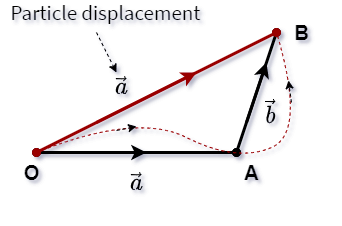So, we can write the resultant vector in this way according to the rules of vector addition.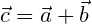That is, according to the above discussion, we can say that the resultant vector is the result of the addition of multiple vectors.

You have to follow two laws to easily represent the addition of vectors.

• Vector Triangel Law
• Vector Parallelogram Law

### Vector Triangel Law

According to this formula, if two sides taken in the order of a triangle indicate the value and direction of the two vectors, the third side taken in the opposite order will indicate the value and direction of the resultant vector of the two vectors.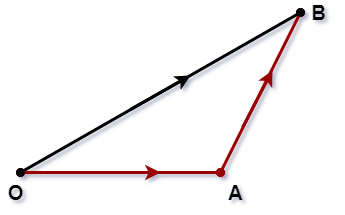That is, if two sides of a triangle rotate clockwise, then the third arm of the triangle rotates counterclockwise. So, take a look at this figure below to understand easily.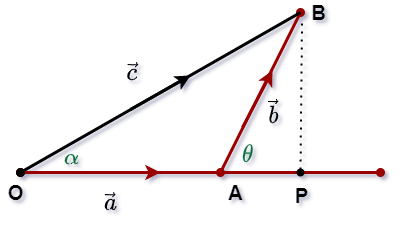As shown in the figure, alpha is the angle between the resultant vector and a vector. And theta is the angle between the vectors a and b. Here c vector is the resultant vector of a and b vectors. And you can write the c vector using the triangle formula

And if you do algebraic calculations, the value of c will be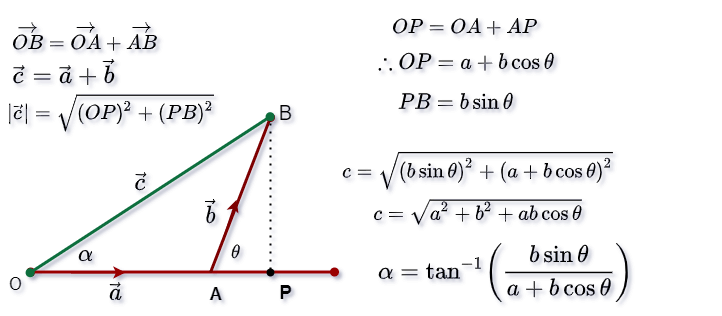So, if you know the absolute value of the two vectors and the value of the intermediate angle, you can easily determine the value of the resolute vector

### Vector Parallelogram Law

The parallelogram of the vector is actually an alternative to the triangle formula of the vector. Thus, the sum of two vectors is also determined using this formula.

If two adjacent sides of a parallelogram indicate the values and directions of two vectors, then the diagonal of the parallelogram drawn by the intersection of the two sides will indicate the values and directions of the resultant vectors.

Suppose, as shown in the figure below, OA and AB indicate the values ​​and directions of the two vectors And OB is the resultant vector of the two vectors. Here α is the angle between the two vectors. And the resultant vector is located at an angle θ with the OA vector.

pic

Thus, the value of the resultant vector will be according to this formula

And the resultant vector is located at an angle OA with the θ vector. That is

### Some Special Cases of vector Addition

The following are some special cases to make vector calculation easier to represent.

1. α=0° : Here α is the angle between the two vectors. That is, if the value of α is zero, the two vectors are on the same side. In this case, the value of the resultant vector will be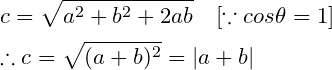Thus, the absolute value of the resultant vector will be equal to the sum of the absolute values of the two main vectors. Notice the image below

pic

2. α=180° : Here, if the angle between the two vectors is 180°, then the two vectors are opposite to each other. That is, the value of cos here will be -1.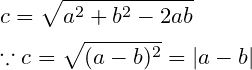so,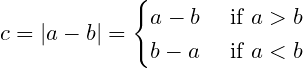Here the absolute value of the resultant vector is equal to the absolute value of the subtraction of the two vectors. And the resultant vector will be oriented towards it whose absolute value is higher than the others.

3. a=b and α=180° : Here the two vectors are of equal value and are in opposite directions to each other. In this case, the absolute value of the resultant vector will be zero. That is, the resolution vector is a null vector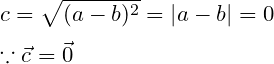2. α=90° : If the angle between the two vectors is 90 degrees. The value of cosθ will be zero. So, here the resultant vector will follow the formula of Pythagoras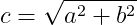In this case, the two vectors are perpendicular to each other. And the resultant vector will be located at the specified angle with the two vectors. Suppose, here two vectors a, b are taken and the resultant vector c is located at angle θ with a vector Then the direction of the resultant vector will be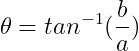## 2. Vector Subtraction

According to the rules of general algebra, subtraction is represented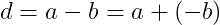Subtracting a number with a positive number gives the same result as adding a negative number of exactly the same number. This same rule applies to vector subtraction.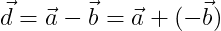That is, the subtraction of vectors a and b will always be equal to the resultant of vectors a and -b. Thus, vector subtraction is a kind of vector addition.

The segments OQ and OS indicate the values ​​and directions of the two vectors a and b, respectively. QO is extended to P in such a way that PO is equal to OQ. As a result, vectors $\vec{OQ}$ and $\vec{OP}$ will be two opposite vectors.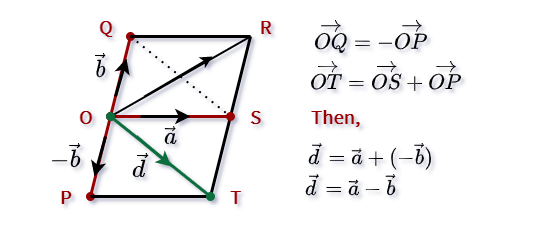When OSTP completes a parallelogram, the OT diagonal represents the result of both a and b vectors according to the parallelogram of the vector. That is, the OT diagonal of the parallelogram indicates the value and direction of the subtraction of the two vectors a and b.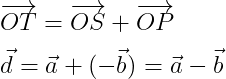## 3. Vector Multiplication (Product by Scalar)

Vector multiplication does not mean dot product and cross product here. Rather, the vector is being multiplied by the scalar.

When you multiply a vector by scalar m, the value of the vector in that direction will increase m times. That is, in the case of scalar multiplication there will be no change in the direction of the vector but the absolute value of the vector will change. So, look at the figure below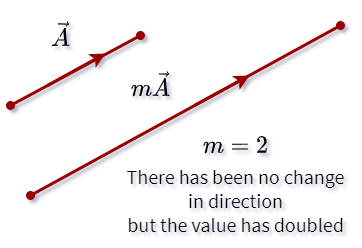So, you can multiply by scalar on both sides of the equation like linear algebra.

Suppose a vector is taken here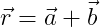And if you multiply by scalar on both sides, the vector will be.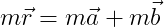Many of you may know the concept of a unit vector. And if you multiply the absolute vector of a vector by the unit vector of that vector, then the whole vector is found. So, notice below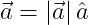In the same way, if a vector has to be converted to another direction, then the absolute value of the vector must be multiplied by the unit vector of that direction.

For example, let us take two vectors a, b. And I want to change the vector of a to the direction of b. In that case, there will be a new vector in the direction of b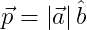## 4. Vector Division

With the help of vector division, you can divide any vector by scalar. For example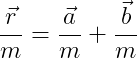You may know that when a unit vector is determined, the vector is divided by the absolute value of that vector.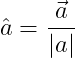## Resolution of Vector (Vector Component) in Physics

Just as it is possible to combine two or more vectors, it is possible to divide a vector into two or more parts. These split parts are called components of a given vector.

If a vector is divided into two or more vectors in such a way that the original vector is the resultant vector of the divided parts. Then those divided parts are called the components of the vector.

Dividing a vector into two components in the process of vector division will solve almost all kinds of problems. Thus, it goes without saying that vector algebra has no practical application of the process of division into many components.

So, below we will discuss how to divide a vector into two components.

### Resolution of vectors in Two Rectangular Components

First, you notice the figure below, where two axial Cartesian coordinates are taken to divide the vector into two components. And the R vector is located at an angle θ with the x-axis.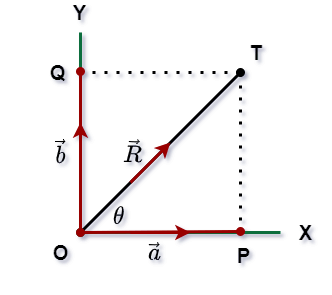And the R vector is divided by two axes OX and OY perpendicular to each other.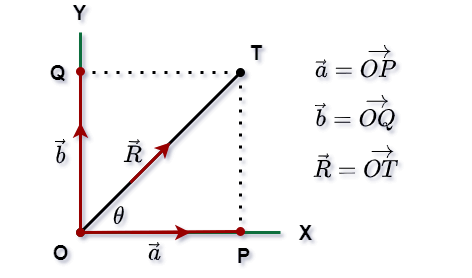According to the given formula,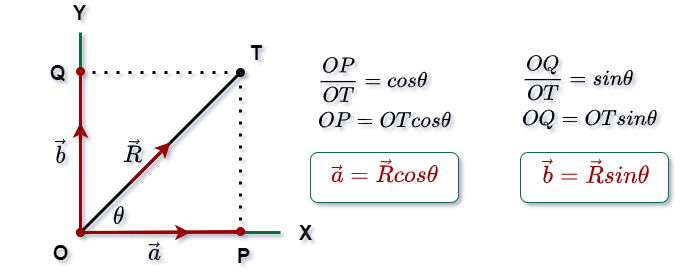Thus, the component along the x-axis of the $\vec{R}$ vector is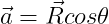And will be the component of the $\vec{R}$ vector along the y-axis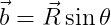## Vector Multiplication(by a vector) in Physics

When you multiply two vectors, the result can be in both vector and scalar quantities.

Thus, based on the result of the vector multiplication, the vector multiplication is divided into two parts.

• Dot Product or Scalar Product
• Cross Product or Vector Product

## 1. Dot Product

Multiplying two vectors produces a scalar. For example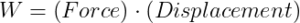Here force and displacement are both vector quantities, but their product is work done, which is a scalar quantity. Such a product is called a scalar product or dot product of two vectors.

And such multiplication is expressed mathematically with a dot(•) mark between two vectors.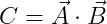So, if two vectors a, b and the angle between them are theta, then their dot product value will be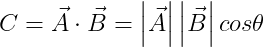The dot product is called a scalar product because the value of the dot product is always in the scalar.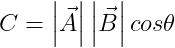### Some properties of scalar product

1. $\vec{A}\cdot \vec{A}=A^{2}$, When Dot Product within the same vector, the result is equal to the square of the value of that vector.

Thus, if the same vector is taken twice, the angle between the two vectors will be zero. Here will be the value of the dot product.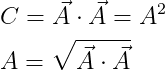2. $\vec{A}\cdot \vec{B}=\vec{A}\cdot \vec{B}$ That is, the scalar product adheres to the exchange rule.

If you move from a to b then the angle between them will be θ. But, in the opposite direction i.e. if you rotate from b to a then the angle will be .3. If two vectors are perpendicular to each other, the scalar product of the two vectors will be zero.

Suppose two vectors a and b are taken here, and the angle between them is θ=90°. then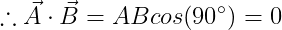4. If the angle between two vectors is θ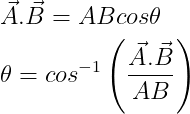6. It is possible to determine the scalar product of two vectors by coordinates.

Let’s say, $\vec{a}=a_{x}\hat{i}+a_{y}\hat{j}+a_{z}\hat{k}$ and $\vec{b}=b_{x}\hat{i}+b_{y}\hat{j}+b_{z}\hat{k}$, that is,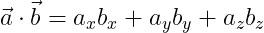## 2. Cross Product

The product of two vectors can be a vector. For Example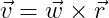Here both the angular velocity and the position vector are vector quantities. And their product linear velocity is also a vector quantity. This type of product is called a vector product.
Such multiplication is expressed mathematically with a cross mark between two vectors. For example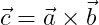Here if the angle between the a and b vectors is θ, you can express the cross product in this way.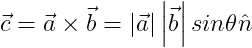Since the result of the cross product is a vector. That is, the direction must always be added to the absolute value of the product. Notice the equation above, n is used to represent the direction of the cross product. That is, here $\hat{n}$ is the perpendicular unit vector with the plane of a, b vector.

Thus, the direction of the cross product will always be perpendicular to the plane of the vectors. So look at this figure below

## Position Vector

Suppose a particle is moving in free space. And you are noticing the location of the particle from the origin of a Cartesian coordinate system. You want to know the position of the particle at a given time.

Suppose the position of the particle at any one time is $(s,y,z)$. According to the vector form, we can write the position of the particle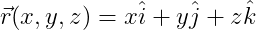So, here $\vec{r}(x,y,z)$ is the position vector of the particle. Because with the help of $\vec{r}(x,y,z)$ you can understand where the particle is located from the origin of the coordinate And which will represent in the form of vectors.

And the distance from the origin of the particle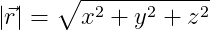When the position of a point in the respect of a specified coordinate system is represented by a vector, it is called the position vector of that particular point.

### Displacement Vector

Suppose a particle is moving from point A to point B. And here the position vectors of points a and b are r1, r2. Then the displacement vector of the particle will be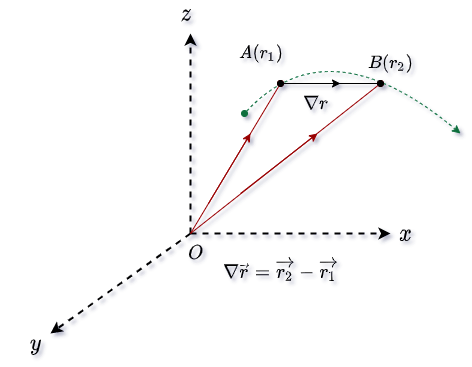Here, if $\vec{r_{1}}=x_{1}\hat{i}+y_{1}\hat{j}+z_{1}\hat{k}$ and $\vec{r_{2}}=x_{2}\hat{i}+y_{2}\hat{j}+z_{2}\hat{k}$, then the displacement vector  $\nabla \vec{r}$ will be